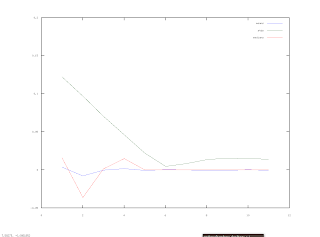## Saturday, 19 June 2010

### Update on recent efforts #3In my last post I spoke about conducting a Monte Carlo test to determine a maximum and minimum slope value for the repeated median straight line fit, the purpose being to establish levels outside of which it could be said that price is unlikely to be moving sideways according to the modelling of prices as a sine wave. A plot of the first step in this procedure is shown above.

The plot shows the MC test output of the slope of the repeated median straight line fit at various multiples of the period of the sine wave model. At 6 on the x-axis, representing a particular multiple, it can be seen that the standard deviation of the test run output is at a minimum, meaning this is the value that sees the least variation between MC runs, and the mean and median of the output at this point is the expected value of zero. This exact pattern repeats for all periods of interest (10 to 50 inclusive). This result is gratifying in that the actual value of the look back period multiplier seems to make sense theoretically, and the fact that it is the same across all periods means that it is a robust parameter. However, because the standard deviation is not zero, the next MC test will be to determine the normalised upper and lower levels already mentioned, using the optimised look back period multiplier represented above.